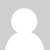### Calculating energy consumption in the Arduino sketch

Gents, I'm trying to figure out how to calculate the energy (KWH) consumption in the Arduino sketch and I'd like to validate my algorithm with you.

According to my research, Energy (KWH) is actually the "integral" of Power over Time, correct (check the figure) ?

This, since we're discrete sampling with the EmonLib, a nice way to calculate a fair approximation is:

energy =  ( ( currentPower + lastPower ) / 2 ) * ( elapsedTime ) / 3600000;

Where:

- energy: will be the the KHW energy consumed between the samples;

- currentPower: apparent power from the current sampling;

- lastPower: apparent power from the last sampling (the one before the current);

- elapsedTime: will be the amount of milliseconds between the two samples;

Is this correct?### Re: Calculating energy consumption in the Arduino sketch

Is this correct? Yes.

If you search the forums, some weeks ago there was a suggestion for a 'better' and more complicated algorithm for calculating the rms values, where we use the 'midpoint' rule http://openenergymonitor.org/emon/node/2412 (not exactly what you are considering but relevant), but if I remember correctly, the conclusion was the difference was less than the margin of error on the original measurements.

There's only one problem with calculating energy in the Arduino: you cannot write the present value into EEPROM each time because of the limit on the number of writes, and if you do not save the value, when you power down or reset the accumulated energy value is lost. This is why we normally accumulate energy in the emonCMS server. I believe someone (possibly Martin Harizanov) has a sketch that, using a mains powered Arduino, senses power loss on the voltage input and writes to EEPROM before the d.c. supply collapses, therefore he has no problem with EEPROM life.

(n.b. It might avoid potential confusion if you use 'present' instead of 'current'  - current = now  or  current = I ? ! ! !)### Re: Calculating energy consumption in the Arduino sketch

Hi Robert, thanks for the reply and clarifications. And yes, current instead of present was a bad idea. ;-)

Yep, my plan is to accumulate Energy in the server side, not on the Arduino.

I'll post the results when I got it all working.

### Re: Calculating energy consumption in the Arduino sketch

I think you'll find the algorithm you have is exactly the one used in emonCMS.